# Intermediate Geometry : How to find the length of the side of a trapezoid

## Example Questions

2 Next →

### Example Question #11 : How To Find The Length Of The Side Of A Trapezoid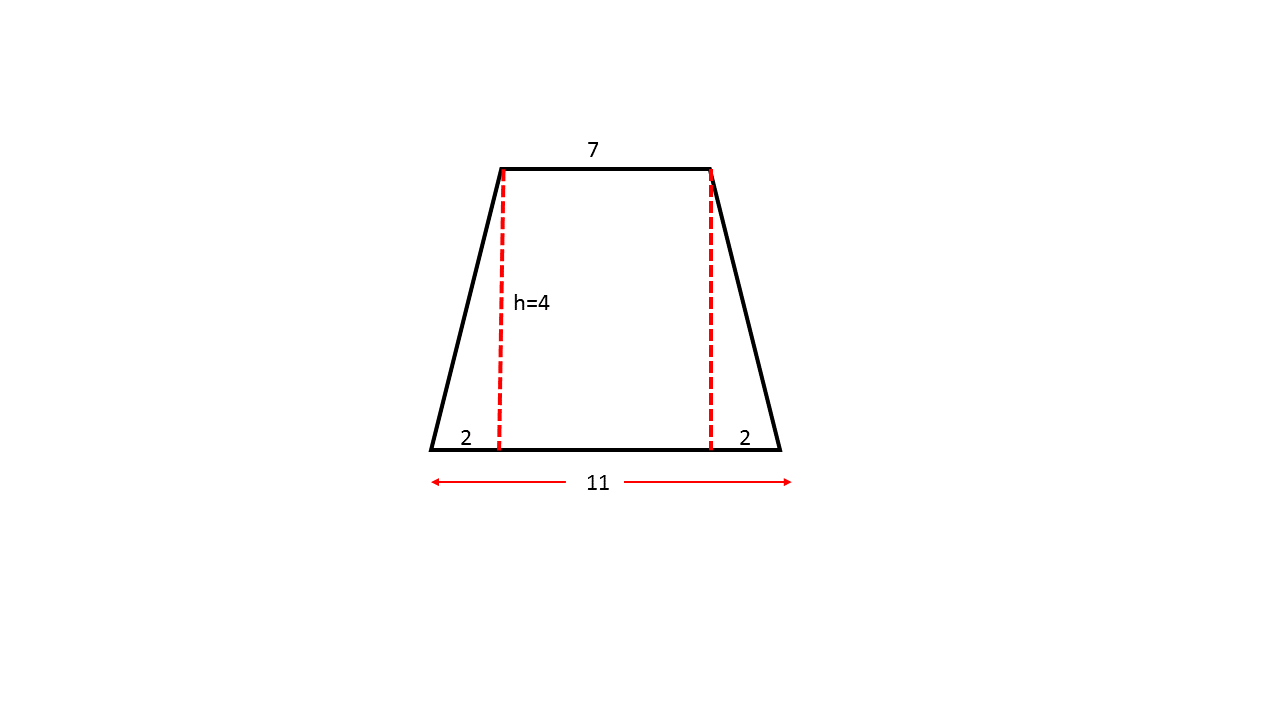Using the isosceles trapezoid shown above, find a possible base measurement for a similar trapezoid where the largest base measurement is.Not enough information is provided.Explanation:

In order for two trapezoids to be similar their corresponding sides must have the same ratio. Since the largest base length in the image isand the corresponding side is, the other base must also betimes greater than the corresponding side shown in the image.

The smaller base shown in the image has a length of, thus the corresponding side in the similar trapezoid must equal: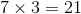### Example Question #11 : Trapezoids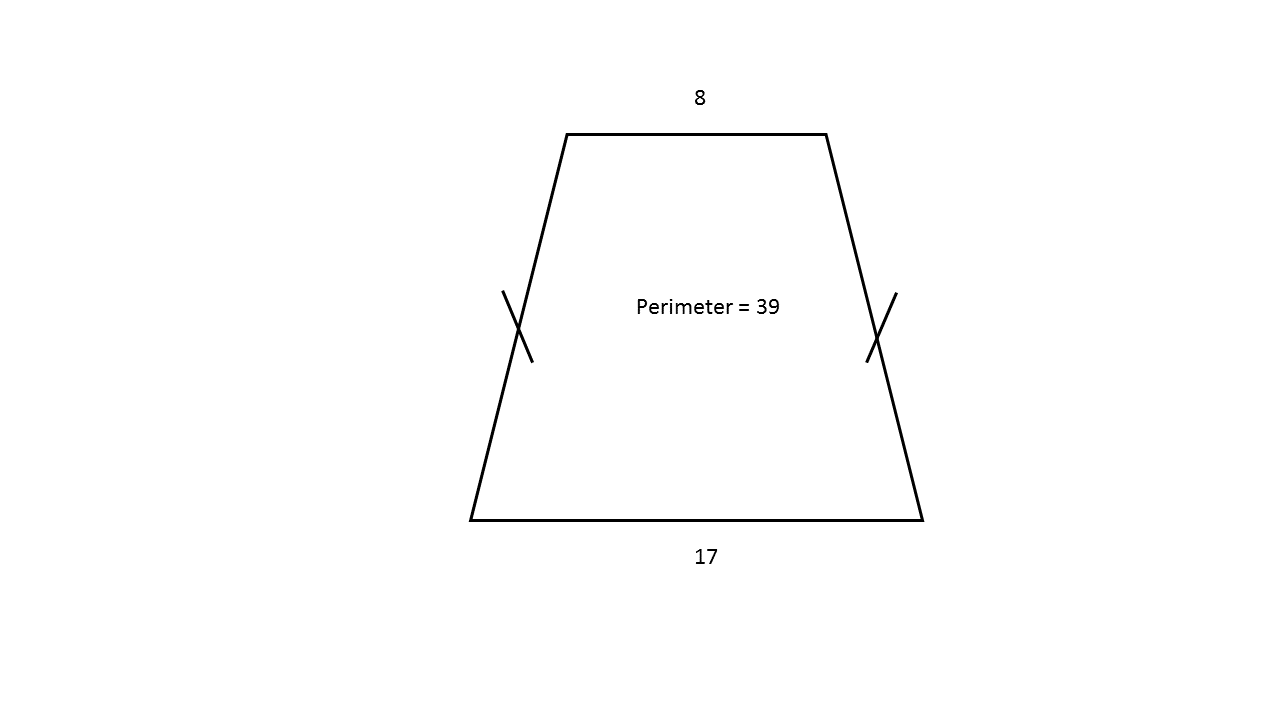Using the image shown above, find the lenght for one of the two nonparallel equivalent sides for a similar trapezoid where the perimeter is equal to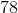Explanation:

In order for two trapezoids to be similar their corresponding sides must have the same ratio. Since the perimeter in the image isand the corresponding perimeter is, the corresponding sides must also betimes greater than the corresponding sides shown in the image.

The nonparallel equivalent sides shown in the image have a length of, thus the corresponding side in the similar trapezoid must equal: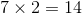### Example Question #13 : Trapezoids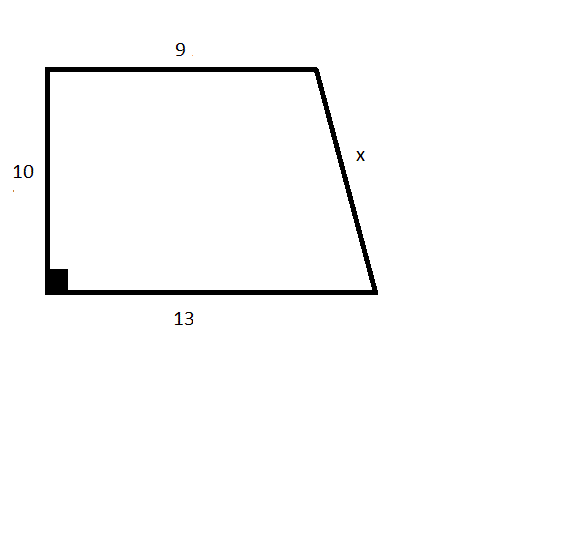Using the trapezoid shown above, find the length of side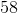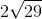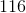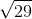Explanation:

In order to find the length of side, first note that the vertical side that has a length ofand the base side with lengthmust be perpendicular because they form a right angle. This means that the height of the trapezoid must equal. A right triangle can be formed on the interior of the trapezoid that has a height ofand a base lenght of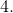The base length can be derived by finding the difference between the two nonequivalent parallel bases.

Thus, the solution can be found by applying the formula: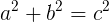, where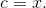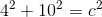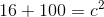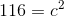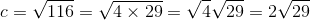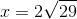### Example Question #14 : Trapezoids

An isosceles trapezoid has base measurements of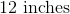and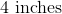. The perimeter of the trapezoid is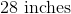. Find the length for one of the two remaining sides.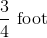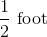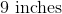Explanation:

To solve this problem, first note that an isosceles trapezoid has two parallel bases that are nonequivalent in length. Additionally, an isosceles trapezoid must have two nonparallel sides that have equivalent lengths. Since this problem provides the length for both of the bases as well as the total perimeter, the missing sides can be found using the following formula: Perimeter= Base one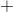Base two(leg), where the length of "leg" is one of the two equivalent nonparallel sides.

Note: once you find the answer in inches, convert that quantity to an equivalent amount in feet.

Thus, the solution is: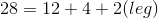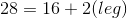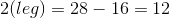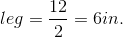inch is equal to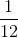foot. Therefore,inches is equvalent tofoot.

### Example Question #13 : Trapezoids

Quadrilateralsuch that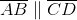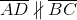and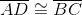. Which of the following correctly describes this quadrilateral?

Rhombus

Rectangle

Isosceles trapezoid

Kite

Non-isosceles trapezoid

Quadrilateralhas at least one pair of parallel sides,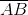and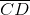. The figure is, by definition, a parallelogram if and only if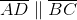, and, by definition, a trapezoid if and only if. Since, the figure is a trapezoid with basesandand legs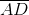and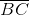. Since, the trapezoid is by definition an isosceles trapezoid.